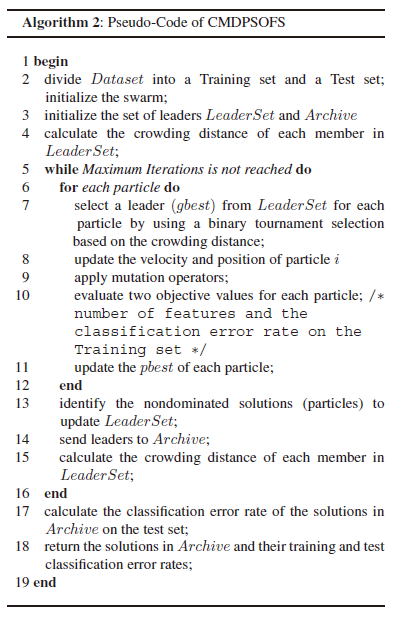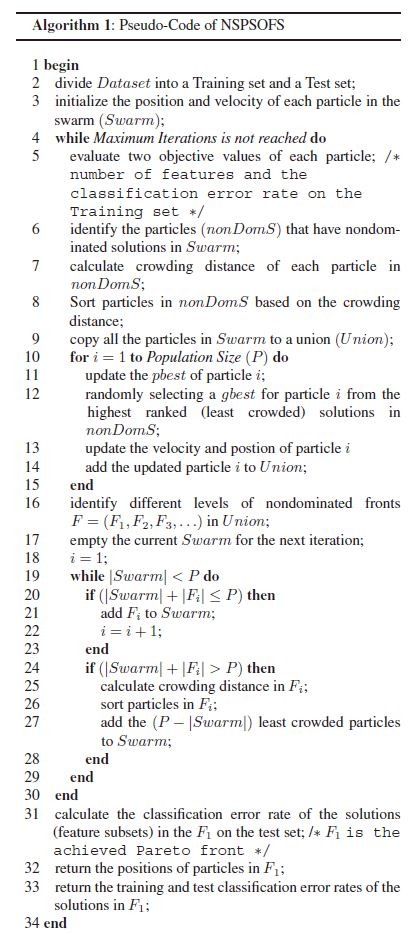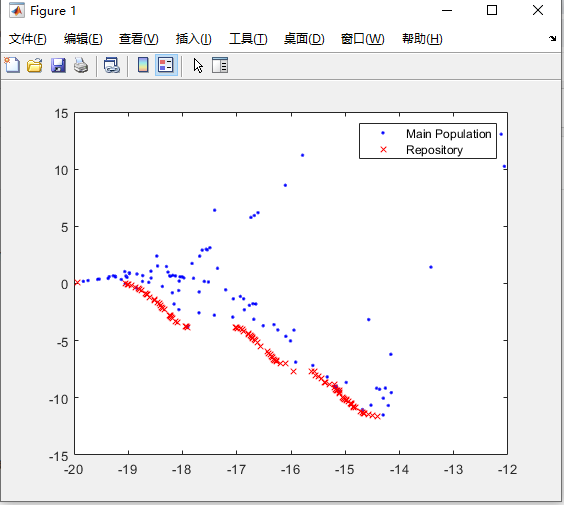• 这一篇是Xue Bing在一区cybernetics发的论文，里面提出了两个多目标PSO特征选择算法，一个是NSPSO另一个是CMDPSO。其中NSPSO是参考了NSGA2的框架和思想。下面具体说说CMDPSO。 CMDPSO全称是Crowding，Mutation，...
这一篇是Xue Bing在一区cybernetics发的论文，里面提出了两个多目标PSO特征选择算法，一个是NSPSO另一个是CMDPSO。其中NSPSO是参考了NSGA2的框架和思想。下面具体说说CMDPSO。
CMDPSO全称是Crowding，Mutation，Dominance PSO算法。
NSPSO算法概述与实现
http://www.omegaxyz.com/2018/09/01/nspso/
http://www.omegaxyz.com/2018/09/22/nspso_matlab/
PSO算法
http://www.omegaxyz.com/2018/01/17/matlab_pso/
个体拥挤距离
在同一层Fk中需要进行选择性排序，按照个体拥挤距离(crowding distance)大小排序。个体拥挤距离是Fk上与i相邻的个体i+1和i-1之间的距离，其计算步骤为：
①对同层的个体距离初始化，令L[i]d=0（表示任意个体i的拥挤距离）。
②对同层的个体按照第m个目标函数值升序排列。
③对于处在排序边缘上的个体要给予其选择优势。
④对于排序中间的个体，求拥挤距离
⑤对于不同的目标函数，重复②到④的步骤，得到个体i的拥挤距离L[i]d，有限选择拥挤距离较大的个体，可以是计算结果在目标空间均匀地分布，维持群体的多样性。
伪代码注意archive中存的是每一代的精英粒子集合
具体流程
①划分数据集
②初始化精英集合和存档Archive
③计算拥挤距离
④二进制锦标赛选择一个一个精英个体来作为gbest指导更新
⑤更新粒子
⑥使用变异算子
⑦粒子真实评价
⑧将LeaderSet加入存档
⑨迭代完成后计算存档中的两个目标值
更多内容请访问：http://www.omegaxyz.com/2018/10/15/cmdpso/


展开全文机器学习 特征选择
• 研究风光互补发电系统优化,提出一种基于多目标微粒群算法的优化配置方法。首先,提出“有效微粒”的概念,在储备集中选择“有效微粒”,进行拥挤距离排序,并采用轮换的方法选择全局引导者;然后,根据“有效微粒”的个数,...
• 这一篇是Xue Bing在一区cybernetics发的论文，里面提出了两个多目标PSO特征选择算法，一个是NSPSO另一个是CMDPSO。其中NSPSO是参考了NSGA2的框架和思想。下面具体说说NSPSO。 非支配排序 来自NSGA2中的非支配排序 ...
这一篇是Xue Bing在一区cybernetics发的论文，里面提出了两个多目标PSO特征选择算法，一个是NSPSO另一个是CMDPSO。其中NSPSO是参考了NSGA2的框架和思想。下面具体说说NSPSO。

非支配排序
来自NSGA2中的非支配排序

该需要保存两个量：

(1).支配个数np。该量是在可行解空间中可以支配个体p的所有个体的数量。

(2).被支配个体集合SP。该量是可行解空间中所有被个体p支配的个体组成的集合。

下面是fast_nondominated_sort的伪代码

个体拥挤距离
在同一层Fk中需要进行选择性排序，按照个体拥挤距离(crowding distance)大小排序。个体拥挤距离是Fk上与i相邻的个体i+1和i-1之间的距离，其计算步骤为：
①对同层的个体距离初始化，令L[i]d=0（表示任意个体i的拥挤距离）。
②对同层的个体按照第m个目标函数值升序排列。
③对于处在排序边缘上的个体要给予其选择优势。
④对于排序中间的个体，求拥挤距离：

（其中：L[i+1]m为第i+1个体的第m目标函数值fmax，fmin分别为集合中第m目标函数的最大和最小值。）
⑤对于不同的目标函数，重复②到④的步骤，得到个体i的拥挤距离L[i]d，有限选择拥挤距离较大的个体，可以是计算结果在目标空间均匀地分布，维持群体的多样性。

伪代码更多内容访问omegaxyz.com
网站所有代码采用Apache 2.0授权
网站文章采用知识共享许可协议BY-NC-SA4.0授权
© 2018 • OmegaXYZ-版权所有 转载请注明出处 
展开全文机器学习
•研究论文
• 对三个目标函数进行优化，如下： function z=MyCost1(x) n=numel(x); z=[0 0]; z(1)=1-exp(-sum((x-1/sqrt(n)).^2)); z(2)=1-exp(-sum((x+1/sqrt(n)).^2)); end function z=MyCost2(x) z1=sum(-10*...
1.问题描述：

对三个目标函数进行优化，如下：

function z=MyCost1(x)

n=numel(x);

z=[0 0];

z(1)=1-exp(-sum((x-1/sqrt(n)).^2));

z(2)=1-exp(-sum((x+1/sqrt(n)).^2));

end

function z=MyCost2(x)

z1=sum(-10*exp(-0.2*sqrt(x(1:end-1).^2+x(2:end).^2)));

z2=sum(abs(x).^0.8+5*(sin(x.^3)));

z=[z1 z2];

end

function z=MyCost3(x)

x(2)=x(2)*60-30;

z1=x(1);

g=11+x(2)^2-10*cos(2*pi*x(2));

if z1<=g
h=1-sqrt(z1/g);
else
h=0;
end

z2=g*h;

z=[z1 z2];

end

2.部分程序：

clc;
clear;
close all;

%% Problem Definition

TestProblem=2;   % Set to 1, 2, or 3

switch TestProblem
case 1
CostFunction=@(x) MyCost1(x);
nVar=5;
VarMin=-4;
VarMax=4;

case 2
CostFunction=@(x) MyCost2(x);
nVar=3;
VarMin=-5;
VarMax=5;

case 3
CostFunction=@(x) MyCost3(x);
nVar=2;
VarMin=0;
VarMax=1;
end

VarSize=[1 nVar];

VelMax=(VarMax-VarMin)/10;

%% MOPSO Settings

nPop=100;   % Population Size

nRep=100;   % Repository Size

MaxIt=100;  % Maximum Number of Iterations

phi1=2.05;
phi2=2.05;
phi=phi1+phi2;
chi=2/(phi-2+sqrt(phi^2-4*phi));

w=chi;              % Inertia Weight
wdamp=1;            % Inertia Weight Damping Ratio
c1=chi*phi1;        % Personal Learning Coefficient
c2=chi*phi2;        % Global Learning Coefficient

alpha=0.1;  % Grid Inflation Parameter

nGrid=10;   % Number of Grids per each Dimension

beta=4;     % Leader Selection Pressure Parameter

gamma=2;    % Extra (to be deleted) Repository Member Selection Pressure

%% Initialization

particle=CreateEmptyParticle(nPop);

for i=1:nPop
particle(i).Velocity=0;
particle(i).Position=unifrnd(VarMin,VarMax,VarSize);

particle(i).Cost=CostFunction(particle(i).Position);

particle(i).Best.Position=particle(i).Position;
particle(i).Best.Cost=particle(i).Cost;
end

particle=DetermineDomination(particle);

rep=GetNonDominatedParticles(particle);

rep_costs=GetCosts(rep);
G=CreateHypercubes(rep_costs,nGrid,alpha);

for i=1:numel(rep)
[rep(i).GridIndex rep(i).GridSubIndex]=GetGridIndex(rep(i),G);
end

%% MOPSO Main Loop

for it=1:MaxIt
for i=1:nPop
rep_h=SelectLeader(rep,beta);

particle(i).Velocity=w*particle(i).Velocity ...
+c1*rand*(particle(i).Best.Position - particle(i).Position) ...
+c2*rand*(rep_h.Position -  particle(i).Position);

particle(i).Velocity=min(max(particle(i).Velocity,-VelMax),+VelMax);

particle(i).Position=particle(i).Position + particle(i).Velocity;

flag=(particle(i).Position<VarMin | particle(i).Position>VarMax);
particle(i).Velocity(flag)=-particle(i).Velocity(flag);

particle(i).Position=min(max(particle(i).Position,VarMin),VarMax);

particle(i).Cost=CostFunction(particle(i).Position);

if Dominates(particle(i),particle(i).Best)
particle(i).Best.Position=particle(i).Position;
particle(i).Best.Cost=particle(i).Cost;

elseif ~Dominates(particle(i).Best,particle(i))
if rand<0.5
particle(i).Best.Position=particle(i).Position;
particle(i).Best.Cost=particle(i).Cost;
end
end

end

particle=DetermineDomination(particle);
nd_particle=GetNonDominatedParticles(particle);

rep=[rep
nd_particle];

rep=DetermineDomination(rep);
rep=GetNonDominatedParticles(rep);

for i=1:numel(rep)
[rep(i).GridIndex rep(i).GridSubIndex]=GetGridIndex(rep(i),G);
end

if numel(rep)>nRep
EXTRA=numel(rep)-nRep;
rep=DeleteFromRep(rep,EXTRA,gamma);

rep_costs=GetCosts(rep);
G=CreateHypercubes(rep_costs,nGrid,alpha);

end

disp(['Iteration ' num2str(it) ': Number of Repository Particles = ' num2str(numel(rep))]);

w=w*wdamp;
end

%% Results

costs=GetCosts(particle);
rep_costs=GetCosts(rep);

figure;

plot(costs(1,:),costs(2,:),'b.');
hold on;
plot(rep_costs(1,:),rep_costs(2,:),'rx');
legend('Main Population','Repository');

3.仿真结论：D-55

展开全文• 基于PSO算法多目标电力系统无功优化，吴璇，王建，随着国民经济的迅速发展，电力系统的经济运行日益受到重视，降低网损，提高电网输电效率和电力系统运行的经济性是电网运行部门面
• 智能优化方法作业 PSO?算法实验报告 课程名称 智能优化方法 作者姓名 专 业 控制工程 目录 第一章? 问题描述. 1 第二章? 算法设计. 1 2.1? 解及目标函数的表达. 1 2.1.1? 种群的编码. 1 2.1.2? 初始种群的产生. 1 ...
• 中长期动态优化配矿是研究如何制定在一个较长时期内采矿顺序与配矿的问题，是一个高度非线性受限条件下的多目标优化问题。采用多轮粒子群算法(particle swarm optimization algorithm, PSO)来求解矿山企业动态配矿...
• 通过对粒子群全局寻优能力和灰色理论的适应性综合分析，研究提出耦合粒子群算法(Particle Swarm Optimization， PSO)和灰色关联(Gray Relevancy Analysis，GRA)的多目标工艺参数优化算法。该方法将多目标函数的优化...
• 将最大最小化适应度函数与罚函数相结合，提出了一种实用有效求解多目标约束优化问题的粒子群算法。采用归类和比较的思想进行替换非劣解；改变以往全局最优值的选取方法，而采用轮序方式从非劣解中获取。实验证明改进...
• 以压制敌方防空火力任务为背景，考虑战场地形与威胁分布、击毁目标需要的火力以及无人机的战斗毁伤概率等因素，建立了架无人机协同攻击个地面目标的任务规划模型，并提出并行遗传粒子群优化算法（GAPSO）求解...
• 对此构建了目标信道设计的多目标函数，提出了求解离散多目标优化问题的粒子群算法（DMOPSO），给出了种群编码、更新方式离散化设计。仿真结果表明，所提出的频谱切换算法得到的最优信道访问解集能够兼顾网络的实时性...信道容量 粒子群优化
• PSO进行进行特征选择其主要思想是：将子集的选择看作是一个搜索寻优问题（wrapper方法），生成不同的组合，对组合进行评价，再与其他的组合进行比较。...更机器学习，MATLAB，Python内容访问omegaxyz.
PSO进行进行特征选择其主要思想是：将子集的选择看作是一个搜索寻优问题（wrapper方法），生成不同的组合，对组合进行评价，再与其他的组合进行比较。这样就将子集的选择看作是一个是一个优化问题。

简单PSO MATLAB代码及概述请见：http://www.omegaxyz.com/2018/01/17/matlab_pso/
更多机器学习，MATLAB，Python内容访问omegaxyz.com
下面是PSO进行特征选择的代码（注意：整体代码是单目标只优化错误率，注意训练使用的是林志仁SVM，数据集是Parkinson，可以到UCI上下载，训练的结果是错误率）

数据集分割为训练集和测试集：

function divide_datasets
load Parkinson.mat;
dataMat=Parkinson_f;
len=size(dataMat,1);
%归一化
maxV = max(dataMat);
minV = min(dataMat);
range = maxV-minV;
newdataMat = (dataMat-repmat(minV,[len,1]))./(repmat(range,[len,1]));

Indices   =  crossvalind('Kfold', length(Parkinson_label), 10);
site = find(Indices==1|Indices==2|Indices==3);
train_F = newdataMat(site,:);
train_L = Parkinson_label(site);
site2 = find(Indices~=1&Indices~=2&Indices~=3);
test_F = newdataMat(site2,:);
test_L =Parkinson_label(site2);
save train_F train_F;
save train_L train_L;
save test_F test_F;
save test_L test_L;
end

主函数PSOFS：

clear;
clc;
format long;
%------给定初始化条件----------------------------------------------
c1=2;             %学习因子1
c2=2;             %学习因子2
w=0.7;            %惯性权重
MaxDT=100;       %最大迭代次数
D=22;             %搜索空间维数（未知数个数）
M=30;             %初始化群体个体数目
bound=1;
%eps=10^(-6);      %设置精度(在已知最小值时候用)
global answer      %最后所有粒子的结果（包括特征与精确度）
answer=cell(M,3);
global choice     %选出的特征个数
choice=0.8;

%------初始化种群的个体(可以在这里限定位置和速度的范围)------------

x=randn(M,D); %随机初始化位置
v=randn(M,D); %随机初始化速度
x(x>bound)=bound;
x(x<-bound)=-bound;
%------先计算各个粒子的适应度，并初始化p(i)和gbest--------------------
divide_datasets();
for i=1:M
p(i)=fitness(x(i,:),i);
y(i,:)=x(i,:);
end
gbest=x(1,:);             %gbest为全局最优

for i=2:M
if(fitness(x(i,:),i)<fitness(gbest,i))
gbest=x(i,:);
end
end

%------进入主要循环，按照公式依次迭代，直到满足精度要求------------
for t=1:MaxDT
for i=1:M
v(i,:)=w*v(i,:)+c1*rand*(y(i,:)-x(i,:))+c2*rand*(gbest-x(i,:));
x(i,:)=x(i,:)+v(i,:);
if fitness(x(i,:),D)<p(i)
p(i)=fitness(x(i,:),i);
y(i,:)=x(i,:);
end
if p(i)<fitness(gbest,i)
gbest=y(i,:);
end
end
end

%------显示计算结果
disp('*************************************************************')
Solution=gbest';
Result=fitness(gbest,i);
disp('*************************************************************')

特征选择评价函数（利用林志仁的SVM进行训练）：

function error = fitness(x,i)
global answer
global choice
load train_F.mat;
load train_L.mat;
load test_F.mat;
load test_L.mat;

inmodel = x>choice;%%%%%设定恰当的阈值选择特征
answer(i,1)={sum(inmodel(1,:))};
model = libsvmtrain(train_L,train_F(:,inmodel));
[predict_label, ~, ~] = libsvmpredict(test_L,test_F(:,inmodel),model,'-q');
error=0;
for j=1:length(test_L)
if(predict_label(j,1) ~= test_L(j,1))
error = error+1;
end
end
error = error/length(test_L);
answer(i,2)={error};
answer(i,3)={inmodel};
end

结果（选出的特征数和错误率）：
特征数：3
错误率：0.1544

更多机器学习，MATLAB，Python内容访问omegaxyz.com

网站文章采用知识共享许可协议BY-NC-SA4.0授权
© 2018 • OmegaXYZ-版权所有 转载请注明出处 
展开全文机器学习 特征选择
• 基于微分进化(DE)算法的快速收敛性和粒子群优化(PSO)算法的搜索多样性，提出一种融合2种算法优点的混合DE-PSO多目标优化算法来求解DEED问题，该算法基于外部存档集和Pareto占优原则，采用自适应参数的DE和PSO双种群...
• 此书为浙江大学的一篇博士学位论文，详细介绍了PSO（粒子群算法）的原理及多目标优化的实现，对PSO感兴趣的读者可参阅此书。
• CT Yu、BY Qu 和 JJ Liang*，“使用环形拓扑解决多模态多目标问题的多目标粒子群优化器”，IEEE 进化计算汇刊。 （DOI：10.1109 / TEVC.2017.2754271），以获取有关此算法的更多说明。 这篇论文也在“MO_Ring_PSO_...matlab
• 为了解决虚拟企业中的任务分配问题，建立了任务分配的多目标决策优化模型。分析了传统的PSO算法，通过设置算法中速度惯性权重和加速度系数的自动调整，以及引入遗传算法中的变异操作，实现了对该算法的改进。基于...
• 很实用 只要下载matlab程序即可用 还包括了生成图像
• 针对控制性能指标不兼容难以选择控制器,提出了一种改进的PSO控制算法....仿真实验显示:改进的PSO算法有更强的鲁棒性和更好的动静态控制品质.研究结果表明该算法用于污水处理系统控制器参数优选的合理性与可用性.
• 针对粒子群优化（PSO）算法易陷入局部最优，发生早熟这一问题，借鉴复杂适应系统（CAS）理论，将混沌和自适应引入到基本PSO中，形成一种双重自适应PSO算法（DAPSO）。该算法在初始化种群时，采用Logisitic方程产生...
• 该项目旨在开发扩展的PSO算法，该算法对于实际工程中的优化问题具有实用价值。 该算法的特点： 1.众多目标 两组代码，为接缝提供选择引线时使用相对密度测量和叠加方法。 2.多重约束 在提供的所有代码中，使用可行...
• 论文中提出，由于PSO中粒子是跟随着群里中最好的粒子快速向一点收敛，因此直接用PSO算法处理多目标优化，很容易收敛于非劣最优域的局部最优解。 论文所提算法思想为： 1.对应于第i个优化子目标函数，粒子群为其...
• 选取过程采用多目标优化原理结合了随机产生100个点的实际值与MAP图线性插值的平均误差以及选定的标定点数量。为提高算法执行效率，对粒子群算法的迭代准则、惯性权重和学习因子进行改进。结果表明，改进后的粒子群...
• 在烧结法氧化铝生产过程中,各种原料进行混合研磨后形成生料浆并储存在...本文首先建立由目标函数和约 束条件所组成的调槽过程数学模型,然后构造惩罚函数来处理调槽过程中的各种约束条件,并采用粒子群算法 (PSO)对模型进
• 粒子群优化matlab算法，可以实现多目标优化。效果好于遗传算法
• 蜻蜓灰狼pso等等matlab算法集合，融合了多目标处理，提供了现成的数据可直接运行
• 多目标优化—粒子群算法PSO） 1 背景 2 算法介绍 3 算法流程 4 例子说明 1 背景： 粒子群算法（ Particle Swarm Optimization, PSO）最早是由Eberhart和Kennedy在1995年提出来的， 粒子群算法的特点是简单易行，......